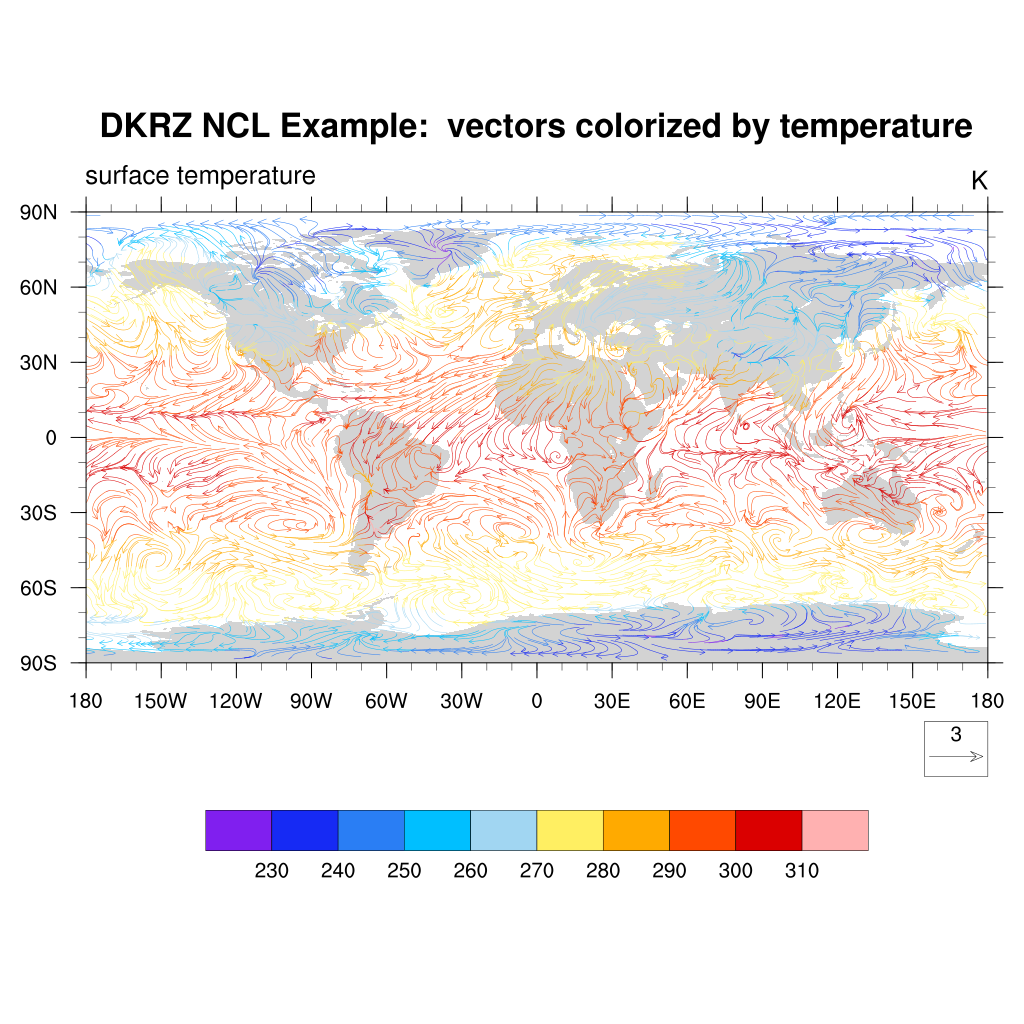# DKRZ NCL vector plot colorized by variable example¶

DKRZ NCL example script:

```;---------------------------------------------------
;  DKRZ NCL Examples:   NCL_vector_plot_colorized.ncl
;
;  30.10.14 kmf
;---------------------------------------------------
;-- define file and variable names
diri  = "./"
fili  = "rectilinear_grid_2D.nc"
uname = "u10"
vname = "v10"
tname = "tsurf"

u   =  f->\$uname\$(0,:,:)                       ;-- first time step
v   =  f->\$vname\$(0,:,:)                       ;-- first time step
t   =  f->\$tname\$(0,:,:)                       ;-- first time step

;-- define the workstation (graphic will be written to a file)
wks =  gsn_open_wks("png","plot_vector_colorized")
gsn_define_colormap(wks,"ncl_default")        ;-- choose color map

;-- set plot resources
res                      =  True
res@gsnMaximize          =  True              ;-- maxmize plot in frame
res@vcMinFracLengthF     =  1.0               ;-- length of min vector as fraction of reference vector.
res@vcRefMagnitudeF      =  3.0               ;-- make vectors larger
res@vcRefLengthF         =  0.045             ;-- ref vec length
res@vcGlyphStyle         = "CurlyVector"      ;-- turn on curly vectors
res@vcMinDistanceF       =  0.01              ;-- thin out vectors
res@tiMainString         = "DKRZ NCL Example:  vectors colorized by temperature" ;-- set title string

;-- draw the vectors
plot = gsn_csm_vector_scalar_map_ce(wks,u,v,t,res)
```

Result: